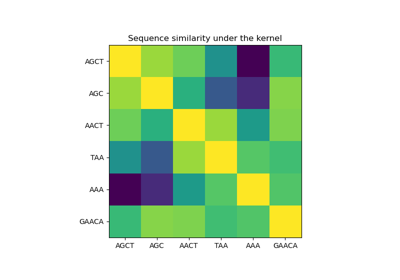# sklearn.gaussian_process.kernels.Hyperparameter¶

class sklearn.gaussian_process.kernels.Hyperparameter(name, value_type, bounds, n_elements=1, fixed=None)[source]

A kernel hyperparameter’s specification in form of a namedtuple.

New in version 0.18.

Attributes:
namestr

The name of the hyperparameter. Note that a kernel using a hyperparameter with name “x” must have the attributes self.x and self.x_bounds

value_typestr

The type of the hyperparameter. Currently, only “numeric” hyperparameters are supported.

boundspair of floats >= 0 or “fixed”

The lower and upper bound on the parameter. If n_elements>1, a pair of 1d array with n_elements each may be given alternatively. If the string “fixed” is passed as bounds, the hyperparameter’s value cannot be changed.

n_elementsint, default=1

The number of elements of the hyperparameter value. Defaults to 1, which corresponds to a scalar hyperparameter. n_elements > 1 corresponds to a hyperparameter which is vector-valued, such as, e.g., anisotropic length-scales.

fixedbool, default=None

Whether the value of this hyperparameter is fixed, i.e., cannot be changed during hyperparameter tuning. If None is passed, the “fixed” is derived based on the given bounds.

Examples

>>> from sklearn.gaussian_process.kernels import ConstantKernel
>>> from sklearn.datasets import make_friedman2
>>> from sklearn.gaussian_process import GaussianProcessRegressor
>>> from sklearn.gaussian_process.kernels import Hyperparameter
>>> X, y = make_friedman2(n_samples=50, noise=0, random_state=0)
>>> kernel = ConstantKernel(constant_value=1.0,
...    constant_value_bounds=(0.0, 10.0))


We can access each hyperparameter:

>>> for hyperparameter in kernel.hyperparameters:
...    print(hyperparameter)
Hyperparameter(name='constant_value', value_type='numeric',
bounds=array([[ 0., 10.]]), n_elements=1, fixed=False)

>>> params = kernel.get_params()
>>> for key in sorted(params): print(f"{key} : {params[key]}")
constant_value : 1.0
constant_value_bounds : (0.0, 10.0)


Methods

 count(value, /) Return number of occurrences of value. index(value[, start, stop]) Return first index of value.
__call__(*args, **kwargs)

Call self as a function.

bounds

Alias for field number 2

count(value, /)

Return number of occurrences of value.

fixed

Alias for field number 4

index(value, start=0, stop=sys.maxsize, /)

Return first index of value.

Raises ValueError if the value is not present.

n_elements

Alias for field number 3

name

Alias for field number 0

value_type

Alias for field number 1

## Examples using sklearn.gaussian_process.kernels.Hyperparameter¶Gaussian processes on discrete data structures

Gaussian processes on discrete data structures